# Mean State

Period Mean (original grids) [Watt m-2]
Model Period Mean (intersection) [Watt m-2]
Model Period Mean (complement) [Watt m-2]
Benchmark Period Mean (intersection) [Watt m-2]
Benchmark Period Mean (complement) [Watt m-2]
Bias [Watt m-2]
RMSE [Watt m-2]
Phase Shift [months]
Bias Score 
RMSE Score 
Seasonal Cycle Score 
Spatial Distribution Score 
Interannual Variability Score 
Overall Score 
Benchmark [-] 122.
CRUNCEPv7 [-] 138. 138. 120. 164. 17.9 26.7 1.56 0.344 0.413 0.779 0.954 0.527 0.572
GSWP3v1 [-] 119. 119. 120. 164. -1.10 17.0 0.910 0.723 0.449 0.877 0.894 0.656 0.675
WFDEI [-] 124. 124. 120. 164. 4.47 22.2 1.50 0.582 0.378 0.779 0.846 0.692 0.609
Period Mean (original grids) [Watt m-2]
Model Period Mean (intersection) [Watt m-2]
Model Period Mean (complement) [Watt m-2]
Benchmark Period Mean (intersection) [Watt m-2]
Benchmark Period Mean (complement) [Watt m-2]
Bias [Watt m-2]
RMSE [Watt m-2]
Phase Shift [months]
Bias Score 
RMSE Score 
Seasonal Cycle Score 
Spatial Distribution Score 
Interannual Variability Score 
Overall Score 
Benchmark [-] 102.
CRUNCEPv7 [-] 113. 113. 101. 165. 12.3 25.4 1.55 0.586 0.461 0.769 0.993 0.574 0.641
GSWP3v1 [-] 103. 102. 101. 165. 1.78 17.9 1.01 0.751 0.528 0.852 0.963 0.633 0.709
WFDEI [-] 116. 115. 101. 165. 14.9 26.5 1.57 0.541 0.469 0.767 0.906 0.667 0.636
Period Mean (original grids) [Watt m-2]
Model Period Mean (intersection) [Watt m-2]
Model Period Mean (complement) [Watt m-2]
Benchmark Period Mean (intersection) [Watt m-2]
Benchmark Period Mean (complement) [Watt m-2]
Bias [Watt m-2]
RMSE [Watt m-2]
Phase Shift [months]
Bias Score 
RMSE Score 
Seasonal Cycle Score 
Spatial Distribution Score 
Interannual Variability Score 
Overall Score 
Benchmark [-] 112.
CRUNCEPv7 [-] 118. 117. 110. 159. 9.37 22.8 1.10 0.628 0.568 0.852 0.945 0.520 0.680
GSWP3v1 [-] 111. 111. 110. 159. 1.79 19.7 1.11 0.756 0.578 0.833 0.885 0.598 0.705
WFDEI [-] 120. 120. 110. 159. 10.6 23.7 1.49 0.595 0.555 0.788 0.867 0.590 0.658
Period Mean (original grids) [Watt m-2]
Model Period Mean (intersection) [Watt m-2]
Model Period Mean (complement) [Watt m-2]
Benchmark Period Mean (intersection) [Watt m-2]
Benchmark Period Mean (complement) [Watt m-2]
Bias [Watt m-2]
RMSE [Watt m-2]
Phase Shift [months]
Bias Score 
RMSE Score 
Seasonal Cycle Score 
Spatial Distribution Score 
Interannual Variability Score 
Overall Score 
Benchmark [-] 43.3
CRUNCEPv7 [-] 12.9 12.9 43.2 44.3 -31.4 35.5 0.307 0.571 0.689 0.980 0.837 0.667 0.739
GSWP3v1 [-] 30.7 30.8 43.2 44.3 -13.8 25.3 0.158 0.784 0.677 0.990 0.849 0.782 0.793
WFDEI [-] 5.09 5.18 43.2 44.3 -38.6 41.9 0.225 0.500 0.694 0.985 0.629 0.810 0.719
Period Mean (original grids) [Watt m-2]
Model Period Mean (intersection) [Watt m-2]
Model Period Mean (complement) [Watt m-2]
Benchmark Period Mean (intersection) [Watt m-2]
Benchmark Period Mean (complement) [Watt m-2]
Bias [Watt m-2]
RMSE [Watt m-2]
Phase Shift [months]
Bias Score 
RMSE Score 
Seasonal Cycle Score 
Spatial Distribution Score 
Interannual Variability Score 
Overall Score 
Benchmark [-] 144.
CRUNCEPv7 [-] 159. 159. 140. 160. 22.3 32.0 1.44 0.333 0.399 0.795 0.857 0.479 0.544
GSWP3v1 [-] 130. 130. 140. 160. -5.43 20.0 1.08 0.623 0.427 0.842 0.875 0.611 0.634
WFDEI [-] 144. 144. 140. 160. 7.56 25.3 1.66 0.479 0.388 0.748 0.940 0.572 0.586
Period Mean (original grids) [Watt m-2]
Model Period Mean (intersection) [Watt m-2]
Model Period Mean (complement) [Watt m-2]
Benchmark Period Mean (intersection) [Watt m-2]
Benchmark Period Mean (complement) [Watt m-2]
Bias [Watt m-2]
RMSE [Watt m-2]
Phase Shift [months]
Bias Score 
RMSE Score 
Seasonal Cycle Score 
Spatial Distribution Score 
Interannual Variability Score 
Overall Score 
Benchmark [-] 62.5
CRUNCEPv7 [-] 42.7 42.7 62.3 75.7 -19.5 31.5 0.270 0.713 0.654 0.981 0.864 0.647 0.752
GSWP3v1 [-] 50.3 50.3 62.3 75.7 -11.8 21.5 0.0870 0.814 0.731 0.994 0.940 0.653 0.810
WFDEI [-] 31.7 31.7 62.3 75.7 -30.3 36.6 0.0766 0.587 0.699 0.995 0.805 0.775 0.760
Period Mean (original grids) [Watt m-2]
Model Period Mean (intersection) [Watt m-2]
Model Period Mean (complement) [Watt m-2]
Benchmark Period Mean (intersection) [Watt m-2]
Benchmark Period Mean (complement) [Watt m-2]
Bias [Watt m-2]
RMSE [Watt m-2]
Phase Shift [months]
Bias Score 
RMSE Score 
Seasonal Cycle Score 
Spatial Distribution Score 
Interannual Variability Score 
Overall Score 
Benchmark [-] 72.7
CRUNCEPv7 [-] 66.5 66.3 72.1 94.9 -4.57 30.2 0.743 0.710 0.572 0.929 0.885 0.622 0.715
GSWP3v1 [-] 62.5 62.5 72.1 94.9 -8.32 21.7 0.373 0.789 0.653 0.971 0.993 0.617 0.780
WFDEI [-] 62.4 62.0 72.1 94.9 -8.87 28.2 0.630 0.740 0.575 0.944 0.904 0.693 0.739
Period Mean (original grids) [Watt m-2]
Model Period Mean (intersection) [Watt m-2]
Model Period Mean (complement) [Watt m-2]
Benchmark Period Mean (intersection) [Watt m-2]
Benchmark Period Mean (complement) [Watt m-2]
Bias [Watt m-2]
RMSE [Watt m-2]
Phase Shift [months]
Bias Score 
RMSE Score 
Seasonal Cycle Score 
Spatial Distribution Score 
Interannual Variability Score 
Overall Score 
Benchmark [-] 109.
CRUNCEPv7 [-] 126. 126. 105. 157. 24.4 36.4 0.953 0.408 0.500 0.888 0.991 0.516 0.634
GSWP3v1 [-] 97.9 97.6 105. 157. -3.56 22.4 0.525 0.733 0.541 0.942 0.971 0.562 0.715
WFDEI [-] 114. 114. 105. 157. 12.4 29.1 0.718 0.581 0.496 0.924 0.951 0.581 0.671
Period Mean (original grids) [Watt m-2]
Model Period Mean (intersection) [Watt m-2]
Model Period Mean (complement) [Watt m-2]
Benchmark Period Mean (intersection) [Watt m-2]
Benchmark Period Mean (complement) [Watt m-2]
Bias [Watt m-2]
RMSE [Watt m-2]
Phase Shift [months]
Bias Score 
RMSE Score 
Seasonal Cycle Score 
Spatial Distribution Score 
Interannual Variability Score 
Overall Score 
Benchmark [-] 41.8
CRUNCEPv7 [-] 7.20 7.57 41.9 41.5 -35.3 37.0 0.327 0.515 0.659 0.978 0.678 0.727 0.702
GSWP3v1 [-] 22.3 22.6 41.9 41.5 -20.1 26.2 0.194 0.687 0.677 0.987 0.934 0.760 0.787
WFDEI [-] -3.26 -2.91 41.9 41.5 -45.5 45.9 0.228 0.424 0.679 0.985 0.337 0.832 0.656
Period Mean (original grids) [Watt m-2]
Model Period Mean (intersection) [Watt m-2]
Model Period Mean (complement) [Watt m-2]
Benchmark Period Mean (intersection) [Watt m-2]
Benchmark Period Mean (complement) [Watt m-2]
Bias [Watt m-2]
RMSE [Watt m-2]
Phase Shift [months]
Bias Score 
RMSE Score 
Seasonal Cycle Score 
Spatial Distribution Score 
Interannual Variability Score 
Overall Score 
Benchmark [-] 96.5
CRUNCEPv7 [-] 99.7 99.6 93.2 138. 7.78 23.6 0.609 0.697 0.647 0.954 0.954 0.572 0.745
GSWP3v1 [-] 94.4 94.3 93.2 138. 2.85 19.9 0.313 0.773 0.674 0.976 0.909 0.600 0.768
WFDEI [-] 102. 102. 93.2 138. 9.25 26.5 0.449 0.660 0.626 0.962 0.955 0.641 0.745
Period Mean (original grids) [Watt m-2]
Model Period Mean (intersection) [Watt m-2]
Model Period Mean (complement) [Watt m-2]
Benchmark Period Mean (intersection) [Watt m-2]
Benchmark Period Mean (complement) [Watt m-2]
Bias [Watt m-2]
RMSE [Watt m-2]
Phase Shift [months]
Bias Score 
RMSE Score 
Seasonal Cycle Score 
Spatial Distribution Score 
Interannual Variability Score 
Overall Score 
Benchmark [-] 64.4
CRUNCEPv7 [-] 61.7 61.8 61.9 90.5 0.559 25.8 0.340 0.797 0.672 0.977 0.862 0.718 0.783
GSWP3v1 [-] 55.7 55.7 61.9 90.5 -4.28 18.6 0.265 0.861 0.747 0.982 0.977 0.623 0.823
WFDEI [-] 46.5 46.6 61.9 90.5 -13.3 27.5 0.323 0.750 0.696 0.979 0.801 0.801 0.787
Period Mean (original grids) [Watt m-2]
Model Period Mean (intersection) [Watt m-2]
Model Period Mean (complement) [Watt m-2]
Benchmark Period Mean (intersection) [Watt m-2]
Benchmark Period Mean (complement) [Watt m-2]
Bias [Watt m-2]
RMSE [Watt m-2]
Phase Shift [months]
Bias Score 
RMSE Score 
Seasonal Cycle Score 
Spatial Distribution Score 
Interannual Variability Score 
Overall Score 
Benchmark [-] 115.
CRUNCEPv7 [-] 72.7 72.7 77.4 133. -3.06 29.7 0.787 0.575 0.565 0.908 0.854 0.550 0.669
GSWP3v1 [-] 67.1 67.1 77.4 133. -8.80 23.3 0.525 0.697 0.601 0.938 0.914 0.614 0.727
WFDEI [-] 68.0 68.0 77.4 133. -8.16 30.5 0.755 0.583 0.560 0.907 0.850 0.646 0.684
Period Mean (original grids) [Watt m-2]
Model Period Mean (intersection) [Watt m-2]
Model Period Mean (complement) [Watt m-2]
Benchmark Period Mean (intersection) [Watt m-2]
Benchmark Period Mean (complement) [Watt m-2]
Bias [Watt m-2]
RMSE [Watt m-2]
Phase Shift [months]
Bias Score 
RMSE Score 
Seasonal Cycle Score 
Spatial Distribution Score 
Interannual Variability Score 
Overall Score 
Benchmark [-] 54.9
CRUNCEPv7 [-] 64.5 64.6 51.3 143. 15.6 32.8 1.48 0.522 0.472 0.800 0.885 0.495 0.608
GSWP3v1 [-] 54.1 54.1 51.3 143. 5.11 25.8 0.874 0.666 0.521 0.890 0.860 0.545 0.667
WFDEI [-] 64.6 64.6 51.3 143. 15.3 31.7 1.14 0.514 0.492 0.847 0.825 0.520 0.615
Period Mean (original grids) [Watt m-2]
Model Period Mean (intersection) [Watt m-2]
Model Period Mean (complement) [Watt m-2]
Benchmark Period Mean (intersection) [Watt m-2]
Benchmark Period Mean (complement) [Watt m-2]
Bias [Watt m-2]
RMSE [Watt m-2]
Phase Shift [months]
Bias Score 
RMSE Score 
Seasonal Cycle Score 
Spatial Distribution Score 
Interannual Variability Score 
Overall Score 
Benchmark [-] 44.9
CRUNCEPv7 [-] 14.0 13.8 44.8 45.6 -32.7 36.2 0.154 0.557 0.696 0.990 0.769 0.646 0.726
GSWP3v1 [-] 26.7 26.6 44.8 45.6 -20.0 26.0 0.120 0.700 0.709 0.992 0.906 0.790 0.801
WFDEI [-] 4.57 4.48 44.8 45.6 -41.6 44.4 0.199 0.474 0.694 0.987 0.582 0.785 0.703
Period Mean (original grids) [Watt m-2]
Model Period Mean (intersection) [Watt m-2]
Model Period Mean (complement) [Watt m-2]
Benchmark Period Mean (intersection) [Watt m-2]
Benchmark Period Mean (complement) [Watt m-2]
Bias [Watt m-2]
RMSE [Watt m-2]
Phase Shift [months]
Bias Score 
RMSE Score 
Seasonal Cycle Score 
Spatial Distribution Score 
Interannual Variability Score 
Overall Score 
Benchmark [-] 134.
CRUNCEPv7 [-] 120. 120. 127. 169. 0.586 26.2 1.09 0.591 0.587 0.868 0.964 0.552 0.692
GSWP3v1 [-] 111. 111. 127. 169. -10.6 22.6 0.670 0.669 0.612 0.917 0.950 0.610 0.728
WFDEI [-] 125. 125. 127. 169. 2.24 24.2 0.958 0.653 0.571 0.877 0.876 0.628 0.696
Period Mean (original grids) [Watt m-2]
Model Period Mean (intersection) [Watt m-2]
Model Period Mean (complement) [Watt m-2]
Benchmark Period Mean (intersection) [Watt m-2]
Benchmark Period Mean (complement) [Watt m-2]
Bias [Watt m-2]
RMSE [Watt m-2]
Phase Shift [months]
Bias Score 
RMSE Score 
Seasonal Cycle Score 
Spatial Distribution Score 
Interannual Variability Score 
Overall Score 
Benchmark [-] 99.6
CRUNCEPv7 [-] 90.2 90.4 97.8 136. -5.59 25.8 0.380 0.812 0.627 0.971 0.805 0.568 0.735
GSWP3v1 [-] 83.8 83.9 97.8 136. -12.3 20.4 0.241 0.783 0.726 0.982 0.986 0.622 0.804
WFDEI [-] 85.1 85.3 97.8 136. -10.6 28.8 0.363 0.793 0.597 0.970 0.827 0.739 0.754
Period Mean (original grids) [Watt m-2]
Model Period Mean (intersection) [Watt m-2]
Model Period Mean (complement) [Watt m-2]
Benchmark Period Mean (intersection) [Watt m-2]
Benchmark Period Mean (complement) [Watt m-2]
Bias [Watt m-2]
RMSE [Watt m-2]
Phase Shift [months]
Bias Score 
RMSE Score 
Seasonal Cycle Score 
Spatial Distribution Score 
Interannual Variability Score 
Overall Score 
Benchmark [-] 72.2
CRUNCEPv7 [-] 53.5 53.7 72.0 77.2 -18.4 29.7 0.310 0.725 0.667 0.979 0.949 0.614 0.767
GSWP3v1 [-] 57.9 58.0 72.0 77.2 -13.9 20.9 0.106 0.783 0.761 0.993 0.996 0.661 0.826
WFDEI [-] 44.6 44.7 72.0 77.2 -27.3 35.0 0.279 0.620 0.685 0.981 0.949 0.838 0.793
Period Mean (original grids) [Watt m-2]
Model Period Mean (intersection) [Watt m-2]
Model Period Mean (complement) [Watt m-2]
Benchmark Period Mean (intersection) [Watt m-2]
Benchmark Period Mean (complement) [Watt m-2]
Bias [Watt m-2]
RMSE [Watt m-2]
Phase Shift [months]
Bias Score 
RMSE Score 
Seasonal Cycle Score 
Spatial Distribution Score 
Interannual Variability Score 
Overall Score 
Benchmark [-] 81.6
CRUNCEPv7 [-] 66.6 67.1 81.1 116. -13.2 25.0 0.559 0.730 0.674 0.957 0.984 0.606 0.771
GSWP3v1 [-] 74.1 74.3 81.1 116. -6.22 19.8 0.313 0.808 0.707 0.977 0.994 0.637 0.805
WFDEI [-] 79.5 79.8 81.1 116. -0.589 27.2 0.451 0.793 0.599 0.967 0.962 0.757 0.779
Period Mean (original grids) [Watt m-2]
Model Period Mean (intersection) [Watt m-2]
Model Period Mean (complement) [Watt m-2]
Benchmark Period Mean (intersection) [Watt m-2]
Benchmark Period Mean (complement) [Watt m-2]
Bias [Watt m-2]
RMSE [Watt m-2]
Phase Shift [months]
Bias Score 
RMSE Score 
Seasonal Cycle Score 
Spatial Distribution Score 
Interannual Variability Score 
Overall Score 
Benchmark [-] 132.
CRUNCEPv7 [-] 151. 152. 131. 178. 21.3 28.1 1.10 0.314 0.418 0.849 0.921 0.499 0.570
GSWP3v1 [-] 124. 124. 131. 178. -6.42 19.7 0.933 0.661 0.395 0.869 0.949 0.604 0.646
WFDEI [-] 135. 135. 131. 178. 4.23 23.2 1.32 0.600 0.348 0.812 0.969 0.572 0.608
Period Mean (original grids) [Watt m-2]
Model Period Mean (intersection) [Watt m-2]
Model Period Mean (complement) [Watt m-2]
Benchmark Period Mean (intersection) [Watt m-2]
Benchmark Period Mean (complement) [Watt m-2]
Bias [Watt m-2]
RMSE [Watt m-2]
Phase Shift [months]
Bias Score 
RMSE Score 
Seasonal Cycle Score 
Spatial Distribution Score 
Interannual Variability Score 
Overall Score 
Benchmark [-] 117.
CRUNCEPv7 [-] 124. 124. 117. 145. 7.66 21.7 0.844 0.706 0.551 0.904 0.927 0.506 0.691
GSWP3v1 [-] 112. 112. 117. 145. -4.78 18.7 0.795 0.810 0.564 0.890 0.992 0.597 0.736
WFDEI [-] 118. 117. 117. 145. 1.05 22.1 1.30 0.760 0.521 0.813 0.912 0.671 0.700
Period Mean (original grids) [Watt m-2]
Model Period Mean (intersection) [Watt m-2]
Model Period Mean (complement) [Watt m-2]
Benchmark Period Mean (intersection) [Watt m-2]
Benchmark Period Mean (complement) [Watt m-2]
Bias [Watt m-2]
RMSE [Watt m-2]
Phase Shift [months]
Bias Score 
RMSE Score 
Seasonal Cycle Score 
Spatial Distribution Score 
Interannual Variability Score 
Overall Score 
Benchmark [-] 95.7
CRUNCEPv7 [-] 82.8 82.4 92.5 124. -9.87 27.1 0.576 0.718 0.662 0.949 0.925 0.508 0.737
GSWP3v1 [-] 91.2 90.5 92.5 124. -3.00 24.9 0.383 0.736 0.684 0.965 0.987 0.556 0.768
WFDEI [-] 89.9 89.4 92.5 124. -4.73 29.7 0.780 0.699 0.641 0.904 0.963 0.576 0.737

# Temporally integrated period mean

BENCHMARK MEAN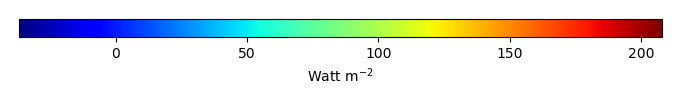MODEL MEANBIAS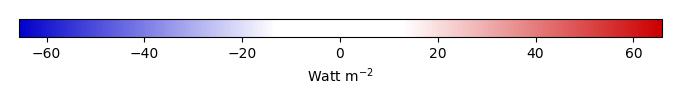BIAS SCORERMSE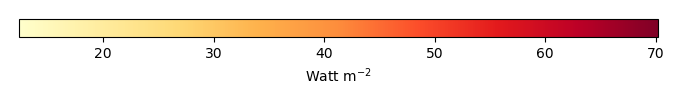RMSE SCOREBENCHMARK INTERANNUAL VARIABILITY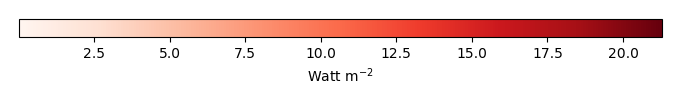MODEL INTERANNUAL VARIABILITYINTERANNUAL VARIABILITY SCOREBENCHMARK MAX MONTHMODEL MAX MONTHDIFFERENCE IN MAX MONTH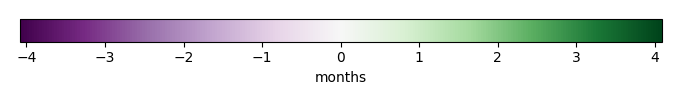SEASONAL CYCLE SCORESPATIAL TAYLOR DIAGRAMMODEL COLORS# Spatially integrated regional mean

MODEL COLORSREGIONAL MEANANNUAL CYCLEMONTHLY ANOMALYANNUAL CYCLE# All Models

BenchmarkCRUNCEPv7GSWP3v1WFDEI# Data Information

creation_date: Tue Jul 1 08:16:58 PDT 2014

source_file: This product is generated from monthly 1 degree GEWEX SRB Radiation observations